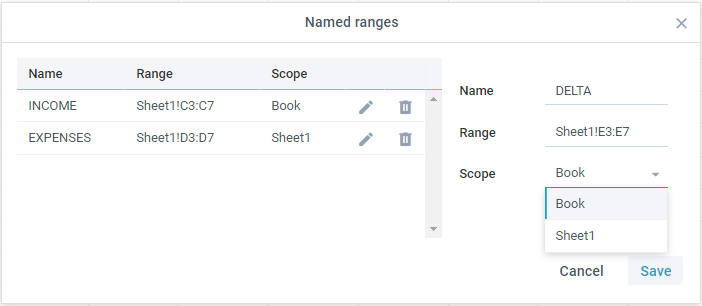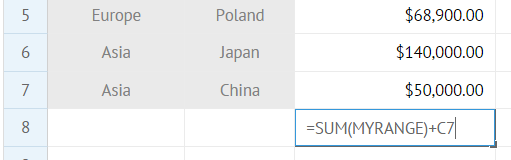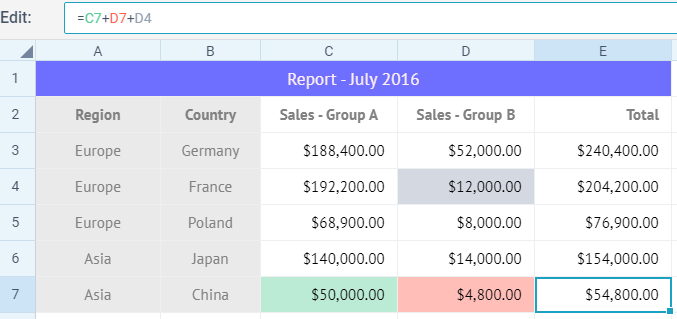# Using Formulas

SpreadSheet allows performing operations with simple cell content (numbers, strings) and operators (arithmetic, comparison, etc.) as well as with built-in functions and custom functions. It is also possible to combine regular operands and functions in more complex formulas. The component processes them and displays the result in the related cells.

## Formula Syntax

The syntax of a formula is the same as in Excel.

1. Formula must start from the '=' sign.
2. The names of functions and cell references must be written in capital letters.
3. The following elements can be used within formulas:
``````"data": [
[ 3, 2, "=A1+B2",""],
[ 4, 2, "=7+8",""],
[ 5, 2, "=7+B2",""],
[ 6, 2, "=SUM(A3+D7)",""]
...
]``````

4 . There are two variants of recording a formula, both produce different results:

• if you want to apply a formula only to two certain cells, you need to specify comma-separated references to these cells, e.g.:
``````"data": [
[ 7, 2, "=SUM(A3,D7)",""]
]``````
• if you want to calculate the values of cells in a range, you should define colon-separated references to the first and the last cells of the range, e.g.:
``````"data": [
[ 7, 2, "=SUM(A3:D7)",""]
]``````

## String Concatenation

You can concatenate string content of cells. There are three possible ways for this:

1 . the & operator with strings or cell references: "=nice"&"trip" or =A1&B2

2 . the + operator with same operands: ="nice"+"trip" or =A1+B2. The "+" operator behaves just as an ordinary JS addition operator (e.g. ="nice"+12).

3 . apply the CONCATENATE() function, like this: =CONCATENATE("nice","trip" or A1,B2). It's also possible to connect strings from a range of cells to produce one continuous text: =CONCATENATE(C2:D4)

## Using Named Ranges in Formulas

You can specify a name for a particular range of cells and then use it in formulas for calculations. Ranges can belong to a particular sheet or the whole Spreadsheet.Thus, the formula will be easier to understand and work with.### Working with ranges via API

You can add a named range and manipulate it through the ranges module:

Used to add new named range globally or to the active/specified sheet. The method takes the following parameters:

• name (string) - range name
• range (string) - range of cells to be included
• scope (string, boolean) - visibility scope.
• Pass a sheet name to add a range visible in this sheet only

To add a range to the active sheet, do not pass the 3rd parameter

``````// add "MYRANGE" range to Sheet 1

Related sample:  Named ranges

## Cross-references in Multiple Sheets

You can link data of several sheets and use these cross references in formulas to process them together.

The rules of creating a reference to an external sheet are quite simple:

• Sheet_Name!Cell_Name - to refer to a cell of other sheet, e.g. =Countries!A4
• Sheet_Name!Cells_Range - to refer to a range of cells from other sheet, e.g. =SUM(Countries!B2:B3)
• Sheet_Name!Named_Range - to refer to a named range from other sheet, e.g. =SUM(Countries!DATA)

Here are some use cases that show how to use cross references in multi sheets:

• provide reference to a cell from a different sheet, e.g. =Data!B8*D13 (where B8 is a cell from the "Data" sheet)
• fill a chart with data from a different sheet, e.g. =SPARKLINE(Countries!DATA,"splineArea","#6666FF") (where "DATA" is a named range from the "Countries" sheet)
• fill a dropdown with a data range from a different sheet, e.g. by referecning the range in the "Add dropdown" dialog as: Countries!NAMES (where "NAMES" is a named range from the "Countries" sheet)

## Formula Editor

• providing the list of possible functions on entering the first letter of the function name in the input;
• entering formula operands either by selecting a range of cells or by typing in the cell reference.It's possible to disable the formula editor, just set the liveEditor property to false in the spreadsheet configuration:

``````webix.ui({